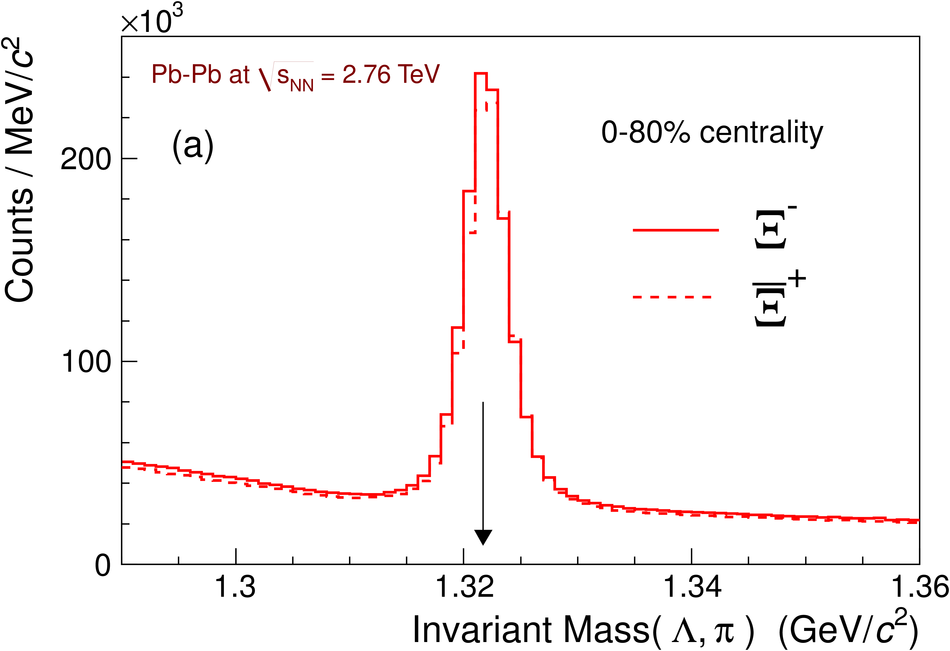# Figure 1

 Invariant mass distributions for $\rm\Xi$ (top) and $\rm\Omega$ (bottom) selected candidates from 0-80% most central Pb-Pb collisions at $\snn=2.76$ TeV. The plots are for candidates in the rapidity interval $|y|< 0.5$ at $\pt>0.6$ and 1.2 GeV/$c$ for $\rm\Xi$ and $\rm\Omega$ candidates, respectively. The arrows point to the PDG mass values.